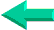### This is an excerpt from Pierre Leclerc (www.excel-vba.com)

The 2 Most Important

Excel Functions AND
Formulas

SUMPRODUCT

AND

INDEX/MATCH

## Lesson 16: ISERROR  Function in Excel

 A B C D 1 Month Unit Price Total Amount Quantity 2 May 365.89 #DIV0 3 Jun 9.95 5698.21 572.6844 4 Mar 32.46 6523.45 200.9689 5 Dec NH 7568.66 #VALUE 6 Nov 0 569.41 #DIV/0 7 Oct 458.65 4569.52 9.962978

The ISERROR function (used with the IF function) will protect you from error messages like the ones above #DIV0 or #VALUE and any other error message generated by a faulty formula. Above the formula in cell D2 is =C2/B2 and the formula has been copied down to D7. The error message #DIV/0 in cell D2 is due to the fact that cell B2 is empty, the error message #VALUE in cell D5 is due to the presence of a non numerical value in cell B5 and the error message in D6 is due to the zero value in cell D5.

The error messages can be replaced using the IFERROR and IF functions. For example:

The formula in D2 is =C2/B2 and if the formula was =IF(ISERROR(C2/B2),0,C2/B2) the result would be 0 instead of the error message #DIV/0. If the formula was =IF(ISERROR(C2/B2),"",C2/B2) the result would be an empty cell. If the formula was =IF(ISERROR(C2/B2),99,C2/B2) the result would be 99 and if the formula was =IF(ISERROR(C2/B2),"Review",C2/B2) the result would be "Review".

The general format is: =IF(ISERROR(the formula to be checked, what if the result is an error,what if the result is not an error ).

Important Note: The IFERROR function was created for Excel 2007. If you share your workbooks with colleagues who use earlier versions of Excel the function ISERROR will not work, it will just generate an error message #NAME. So use the formula IF/ISERROR function instead as it works in all versions of Excel.

SUMPRODUCT  ¦  INDEX/MATCH  ¦  SUBTOTAL  ¦  ISERROR  ¦  HLOOKUP  ¦  LOOKUP  ¦  VLOOKUP
AVERAGEIF  ¦  AVERAGEIFS ¦  COUNTIF  ¦  COUNTIFS  ¦  SUMIF  ¦  SUMIFS  ¦  IFERRORBack home